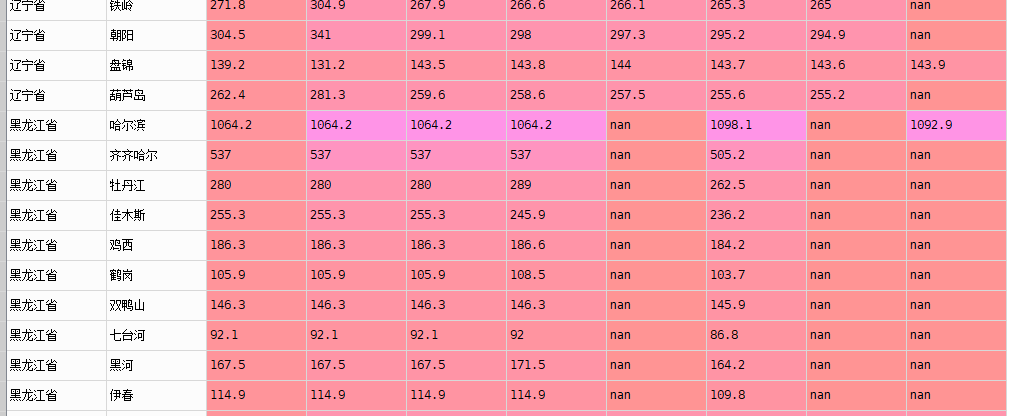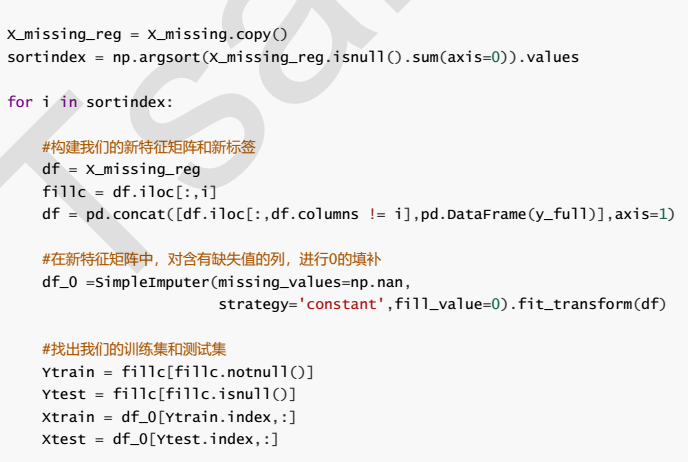• 填补缺失值，前一列向后填充 def fillother(a): b = a.fillna(0) w = [] #防止列索引格式不一致 for m in b.columns: m = str(m) w.append(m) #b.columns = ['省', '市', 2010, 2011, 2012, 2013, ...填补缺失值，前一列向后填充

def fillother(a):
b = a.fillna(0)
w = []
#防止列索引格式不一致
for m in b.columns:
m = str(m)
w.append(m)
#b.columns = ['省', '市', 2010, 2011, 2012, 2013, 2014, 2015, 2016, 2017]
list1 = list(b.columns[2:])
for i in range(len(list1)):
if i ==0:
continue
s = list1[i]
col = b[str(s)]
for index,j in enumerate(col):
if j ==0:
ss = list1[i-1]
v = b[str(ss)][index]
b[str(s)][index] = v
b.to_csv('./'+a+'.csv',index = False)

展开全文• 1、给定一个数据集...利用“全局常量”、“均值或者中位数”来填充缺失值。noise-data-1.txt：5.1 3.5 1.4 0.24.9 3 1.4 0.24.7 3.2 1.3 0.24.6 3.1 1.5 0.25 3.6 1.4 0.25.4 3.9 1.7 0.44.6 3.4 1.4 0.35 3.4 1...
1、给定一个数据集noise-data-1.txt，该数据集中保护大量的缺失值(空格、不完整值等)。利用“全局常量”、“均值或者中位数”来填充缺失值。noise-data-1.txt：5.1 3.5 1.4 0.24.9 3 1.4 0.24.7 3.2 1.3 0.24.6 3.1 1.5 0.25 3.6 1.4 0.25.4 3.9 1.7 0.44.6 3.4 1.4 0.35 3.4 1.5 0.24.4 2.9 1.4 0.24.9 -3.1 1.5 0.15.4 3.7 1.5 0.24.8 3.4 1.6 0.24.8 3 -1.4 0.14.3 3 1.1 0.15.8 4 1.2 0.25.7 4.4 1.5 0.45.4 3.9 1.3 0.45.1 3.5 1.4 0.35.7 3.8 1.7 0.35.1 3.8 -1.5 0.35.4 3.4 1.7 0.25.1 3.7 1.5 0.44.6 3.6 1 0.25.1 3.3 1.7 0.54.8 3.4 1.9 0.2解题思路：首先读入数据，对数据进行处理，去掉空行，利用 “均值来填充缺失值，本题利用Python语言实现，代码如下：import numpy as npdata = []my_list = []con=0noise_data = open('noise-data-1.txt')clean_data = open("clean_data3.txt", 'w')for line in noise_data.readlines():if len(line) == 0:breakif line.count('\n') == len(line):continuedataline =line.strip().split('\t')my_list.append(dataline)con+=1for i in range(0,con):for j in range(0,len(my_list[i])):if my_list[i][j].count('.')==0:miss_row=[]for a in range(0,len(my_list[i])):if float(my_list[i][a])<0:miss_row.append(-float(my_list[i][a]))miss_row.append(float(my_list[i][a]))my_average=round(np.average(miss_row),1)my_list[i][j]=my_averageelse:if float(my_list[i][j])<0:my_list[i][j]=-float(my_list[i][j])my_list[i][j]=float(my_list[i][j])print my_listdef file_write(filename,data_list):file1=open(filename,'w')for i in data_list:for j in i:if type(j)!=str:j=str(j)file1.write(j)file1.write(' ')file1.write('\n')file1.close()return file1filename='clean_data.txt'file_write(filename,my_list)运行结果如下：以上这篇python实现数据预处理之填充缺失值的示例就是小编分享给大家的全部内容了，希望能给大家一个参考，也希望大家多多支持脚本之家。
展开全文• 随机森林填充缺失值 前言： 任何回归都是从特征矩阵中学习，然后求解连续性标签y的值，之所以能实现这个过程，是因为回归算法认为，特征矩阵和标签之前存在着某种关系，实际上特征和标签是可以相互转化的，比如说用...
随机森林填充缺失值
前言：
任何回归都是从特征矩阵中学习，然后求解连续性标签y的值，之所以能实现这个过程，是因为回归算法认为，特征矩阵和标签之前存在着某种关系，实际上特征和标签是可以相互转化的，比如说用地区，环境，附近学校数量预测房价的问题，我们既可以用地区，环境，附近学校数量的数据来预测房价，也可以反过来，用环境，附近学校数量和房价来预测地区，而回归填补缺失值，正式利用了这种情况。
非常使用与一个特征大量数据缺失，其他特征却很完整的情况。
def fill_missing_rf(X,y,to_fill):

"""
使用随机森林填补一个特征的缺失值的函数

参数：
X：要填补的特征矩阵
y：完整的，没有缺失值的标签
to_fill：字符串，要填补的那一列的名称
"""

#构建我们的新特征矩阵和新标签
df = X.copy()
fill = df.loc[:,to_fill]
df = pd.concat([df.loc[:,df.columns != to_fill],pd.DataFrame(y)],axis=1)

# 找出我们的训练集和测试集
Ytrain = fill[fill.notnull()]#特征不缺失的值
Ytest = fill[fill.isnull()]#特征缺失的值
Xtrain = df.iloc[Ytrain.index,:]#特征不缺失的值对应其他n-1个特征+本来的标签
Xtest = df.iloc[Ytest.index,:]#特征缺失的值对应其他n-1个特征+本来的标签

#用随机森林回归来填补缺失值
from sklearn.ensemble import RandomForestRegressor as rfr
rfr = rfr(n_estimators=100)
rfr = rfr.fit(Xtrain, Ytrain)
Ypredict = rfr.predict(Xtest)

return Ypredict

X = data.iloc[:,1:]
y = data["SeriousDlqin2yrs"]#y = data.iloc[:,0]
X.shape#(149391, 10)

#=====[TIME WARNING:1 min]=====#
y_pred = fill_missing_rf(X,y,"MonthlyIncome")

#注意可以通过以下代码检验数据是否数量相同
# y_pred.shape ==  data.loc[data.loc[:,"MonthlyIncome"].isnull(),"MonthlyIncome"].shape

#确认我们的结果合理之后，我们就可以将数据覆盖了
data.loc[data.loc[:,"MonthlyIncome"].isnull(),"MonthlyIncome"] = y_pred

data.info()

问题：对于一个特征缺失，其他特征也有缺失值怎么办？
答案是遍历所有的特征，从缺失最少的特征开始填补（因为填补缺失值最少的特征所需要的准确信息最少）。填补一个特征时，先将其他特征的缺失值用0代题，没完成一次回归预测，就将预测值放到原本的特征矩阵中，再继续填补下一个特征，每一次填补完毕，有缺失值的特征会减少一个，所以每次循环后，需要用0填补的特征就越来越少，当进行到最后一个特征时（这个特征应该时所有特征缺失值最多的），已经没有其他特征需要用0来进行填补，遍历所有的特征后，数据就完整，不再有缺失值。展开全文• 下面小编就为大家分享一篇python实现数据预处理之填充缺失值的示例。具有很好的参考价值，希望对大家有所帮助。一起跟随小编过来看看吧
• 今天小编就为大家分享一篇pandas 使用均值填充缺失值列的小技巧分享，具有很好的参考价值，希望对大家有所帮助。一起跟随小编过来看看吧
• 1、给定一个数据集...利用“全局常量”、“均值或者中位数”来填充缺失值。noise-data-1.txt：5.1 3.5 1.4 0.24.9 3 1.4 0.24.7 3.2 1.3 0.24.6 3.1 1.5 0.25 3.6 1.4 0.25.4 3.9 1.7 0.44.6 3.4 1.4 0.35 3.4 1...
1、给定一个数据集noise-data-1.txt，该数据集中保护大量的缺失值(空格、不完整值等)。利用“全局常量”、“均值或者中位数”来填充缺失值。noise-data-1.txt：5.1 3.5 1.4 0.24.9 3 1.4 0.24.7 3.2 1.3 0.24.6 3.1 1.5 0.25 3.6 1.4 0.25.4 3.9 1.7 0.44.6 3.4 1.4 0.35 3.4 1.5 0.24.4 2.9 1.4 0.24.9 -3.1 1.5 0.15.4 3.7 1.5 0.24.8 3.4 1.6 0.24.8 3 -1.4 0.14.3 3 1.1 0.15.8 4 1.2 0.25.7 4.4 1.5 0.45.4 3.9 1.3 0.45.1 3.5 1.4 0.35.7 3.8 1.7 0.35.1 3.8 -1.5 0.35.4 3.4 1.7 0.25.1 3.7 1.5 0.44.6 3.6 1 0.25.1 3.3 1.7 0.54.8 3.4 1.9 0.2解题思路：首先读入数据，对数据进行处理，去掉空行，利用 “均值来填充缺失值，本题利用Python语言实现，代码如下：import numpy as npdata = []my_list = []con=0noise_data = open('noise-data-1.txt')clean_data = open("clean_data3.txt", 'w')for line in noise_data.readlines():if len(line) == 0:breakif line.count('\n') == len(line):continuedataline =line.strip().split('\t')my_list.append(dataline)con+=1for i in range(0,con):for j in range(0,len(my_list[i])):if my_list[i][j].count('.')==0:miss_row=[]for a in range(0,len(my_list[i])):if float(my_list[i][a])<0:miss_row.append(-float(my_list[i][a]))miss_row.append(float(my_list[i][a]))my_average=round(np.average(miss_row),1)my_list[i][j]=my_averageelse:if float(my_list[i][j])<0:my_list[i][j]=-float(my_list[i][j])my_list[i][j]=float(my_list[i][j])print my_listdef file_write(filename,data_list):file1=open(filename,'w')for i in data_list:for j in i:if type(j)!=str:j=str(j)file1.write(j)file1.write(' ')file1.write('\n')file1.close()return file1filename='clean_data.txt'file_write(filename,my_list)运行结果如下：以上这篇python实现数据预处理之填充缺失值的示例就是小编分享给大家的全部内容了，希望能给大家一个参考，也希望大家多多支持我们。本文标题: python实现数据预处理之填充缺失值的示例本文地址: http://www.cppcns.com/jiaoben/python/215490.html
展开全文• 在使用python里的pandas库进行数据分析工作时，很多时候我们都会遇到这样一个问题：数据缺失。这也是大部分数据分析...这一次我们来说说如何使用fillna()函数填充缺失值。（2）填充（fillna） 函数: DataFrame.fil...
• 点赞、关注再看，养成良好习惯Life is short, U need Python初学Python，...但是如果缺失值占比比较大，这种直接删除缺失值的处理方法就会丢失重要信息。直接删除法处理缺失值时，需要检测样本总体中缺失值的个数。P...
• sparksql_填充缺失值 #为none值填充新值 means = df_miss_no_income.agg(*[fn.mean(c).alias(c) for c in df_miss_no_income.columns if c != 'gender'])\ ....
• python_通过KNN来填充缺失值 # 加载库 import numpy as np from fancyimpute import KNN from sklearn.preprocessing import StandardScaler from sklearn.datasets import make_blobs # 创建模拟特征矩阵 features...
• 也可以使用scikit-learn填充缺失值：使用scikit-learn中的SimpleImputer(原sklearn.preprocessing.Imputer)处理缺失值 函数原型以及参数说明 用法举例 >>> df = pd.DataFrame([[np.nan, 2, np.nan, 0...
• 随机森林填充缺失值优点 随机森林填补通过构造多棵决策树对缺失值进行填补，使填补的数据具有随机性和不确定性，更能反映出这些未知数据的真实分布； 由于在构造决策树过程中，每个分支节点选用随机的部分特征而...机器学习 数据分析 算法
• 处理思路：预测填充缺失值 因为涉及到跟时间有关，技术太菜，不会利用LSTM或者RNN等来预测缺失的数据值，于是就想到KNN算法的设计思想，将所有的指标都当做距离计算的一部分，这里的时间由于是2020-01-02 20:00:00的...python 数据分析 机器学习
• 1、给定一个数据集noise-data-1.txt，该数据集中保护大量的缺失值（空格、不完整值等）。利用“全局常量”、“均值或者中位数”来填充缺失值数据 python 数据预处理
• The implicit conversion of a Python sequence of variable-length lists into a NumPy array cause the array to be of type object.v = [, [1, 2]]np.array(v)>>> array([, [1, 2]], dtype=object...
• pyhton_使用插值法填充缺失值 # 라이브러리를 임포트합니다. import pandas as pd import numpy as np ​ # 날짜를 만듭니다. time_index = pd.date_range("01/01/2010", periods=5, freq="M") ​ # 设置索引 ...
• 数据预处理：PySpark 的实现线性插值填充缺失值 1. Python 实现线性插值填充缺失值 实现函数为： def linear_insert(x1, y1, x2, y2, insert_x): if type(insert_x) == int: insert_x = [insert_x] k = (y2 - y1)...python pyspark 数据挖掘
• 数据预处理：PySpark 的实现线性插值填充缺失值1. Python 实现线性插值填充缺失值实现函数为：def linear_insert(x1, y1, x2, y2, insert_x):if type(insert_x) == int:insert_x = [insert_x]k = (y2 - y1) / (x2 - ...
• 本篇博客分享如何用Python查看、删除、填充缺失值 查看缺失值： df.info() df['col_name'].isnull() #但数据量多时用这条看不清楚... 删除缺失值： df.dropna(thresh=len(df)*0.8, axis=1) #thresh参数含义，...python
• 用随机整数填充缺失值Input an integer value and we have to pad the value by Zeros in C. 输入一个整数值，我们必须在C中用零填充该值。 Example: 例： Input: Enter an integer value: 12 Output: ...
• 缺失值是指数据集中的某些观测存在遗漏的指标值，缺失值的存在同样会影响到数据分析和挖掘的结果。一般而言，当遇到缺失值是可以采三种方法处置：删除法，替换法和插补法。1.删除法使用情况：当确实的观测比例非常低...
• # 缺失值是指数据集中的某些观察存在遗漏的指标值，缺失值的存在同样会影响到数据剖析和挖掘的效果。# 可以采三种方式处置：删除法，替换法和插补法。# 1.删除法使用情况：当确实的观察比例异常低是，如5%以内，可以...
• 删除法如果数据中某个变量大部分都是缺失值，可以考虑删除变量如果缺失值是随机分布的，且缺失的数量并不是很多，可以考虑删除这些缺失的观测值替补法对于连续型变量，如果变量的分布近似或就是正态分布的话，可以用...
• 当我们拿到一份原始数据，首先会查看数据的基础信息，例如每一列数量统计，内存消耗、哪些列有缺失值等。完整的数据对于我们的数据分析工作来说意义重大，但对于实际收集数据的人却不是，所以我们经常会遇到数据缺失...
• 将dataframe中的NAN进行填充填充方法如下： 用0填充： df.fillna(0) 用字符串填充： df.fillna("missing") 用前一个数据填充： df.fillna(method="pad") 用后一个数据代替NaN: df.fillna(method="bfill") ...python
• 机器学习样本理想的情况下倒是不需要做太多处理，但是实际样本很有可能会有很多缺失值，这个时候如果不对缺失值做处理，就根本无法进行模型的训练。例子所用的测试数据来源:使用MLDataTable加载训练数据import Cocoa...
• 1 数据清洗1.1 处理缺失数据对于数值型数据，分为缺失值(NAN)和非缺失值，对于缺失值的检测，可以通过Python中pandas库的Series类对象的isnull方法进行检测。import pandas as pdimport numpy as npstring_data = pd...
• 当数据中存在NaN缺失值时，我们可以用其他数值替代NaN，主要用到了DataFrame.fillna()方法，下面我们来看看具体的用法： 1.先来创建一个带有缺失值的DataFrame: import pandas as pd import numpy as np df=pd....python 数据分析...